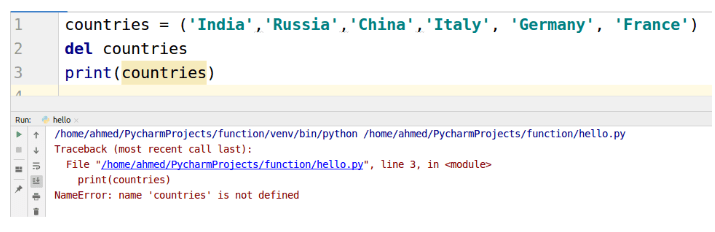Python Tutorials

# What is Python TUPLE ?

## Python TUPLE - Pack, Unpack, Compare, Slicing, Delete, Key

As you know a variable is used to store data like ‘1’,’a’,’bob’, etc. When you have to store all of them belonging to different data types at one place tuple is used. Python tuples are immutable. The main difference between tuples and list is Python is mutability. Lists are mutable while tuples are immutable.

## Declaration

Tuples can hold all types of values and are declared using parentheses. Let’s see how to declare tuples in Python using pycharm.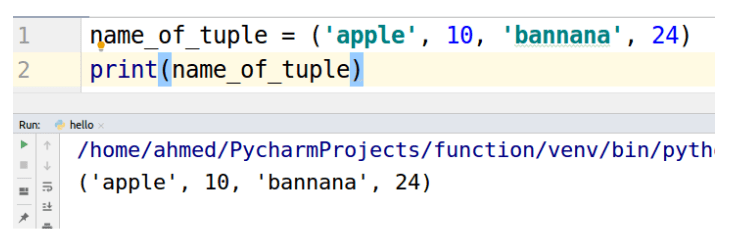## Accessing elements of Tuple

Tuple elements are accessed using the square bracket ‘[]’ operator. Let’s take a look at the example.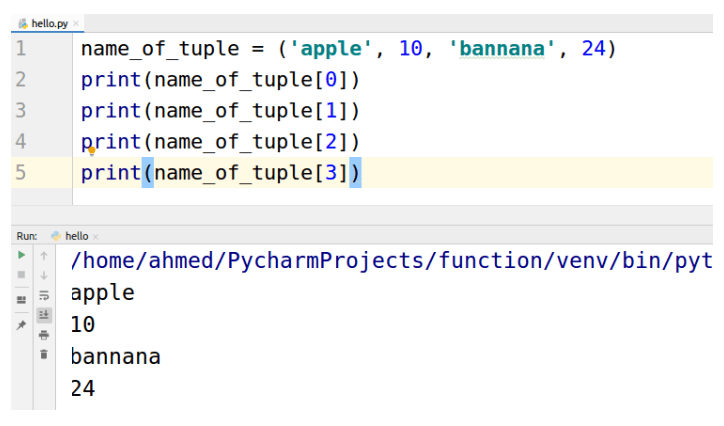We can also specify a range in the square bracket operator using colon “:” to access elements in specific range. Let’s take an example of using colon in square brackets.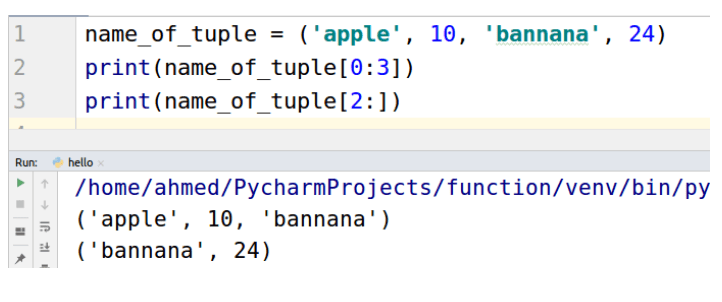## Packing elements into tuple

Packing is placing values in the tuple just like we did in the declaration. Let us see again using another example.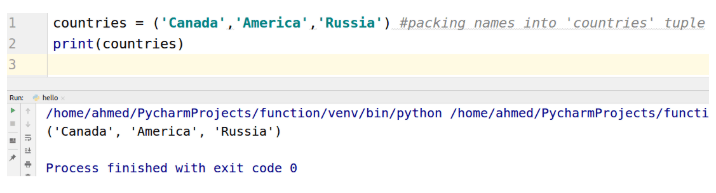## Unpacking elements into variables

Unpacking is the opposite of packing. In unpacking we assign the elements in the tuple to variables. Let us see an example.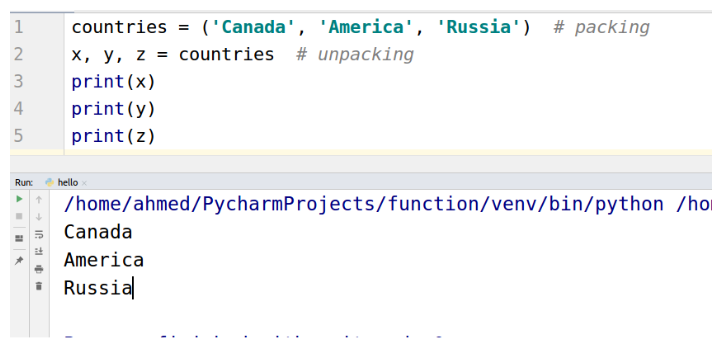## Comparing the tuples

Two or more tuples can be compared using comparison operators. There are major three types of comparisons.

• Equal to ‘==’
• Greater than ‘>’
• Less than ‘<’

Equal is as simple as his name. Let’s take an example.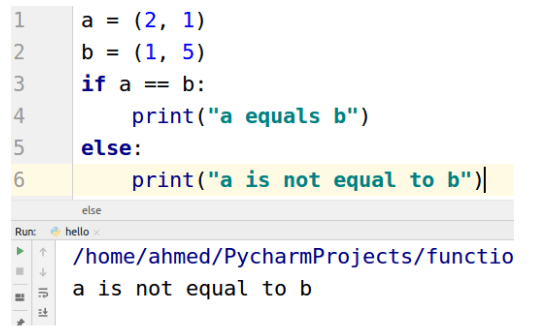Let see an example when a is equal to b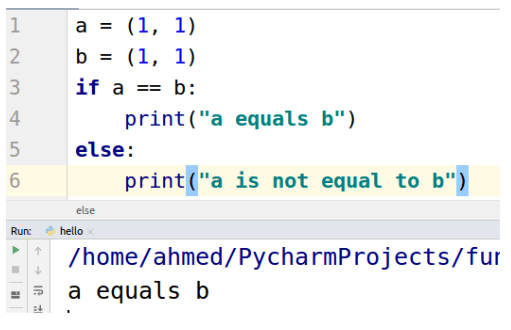“Greater then” and “Less than” is decided from first value to the last value. For example, (0,1) is less than (1,0) and (2,0) is greater than (1, 9). If the first elements are the same then the next values are compared. Let’s take a look at an example.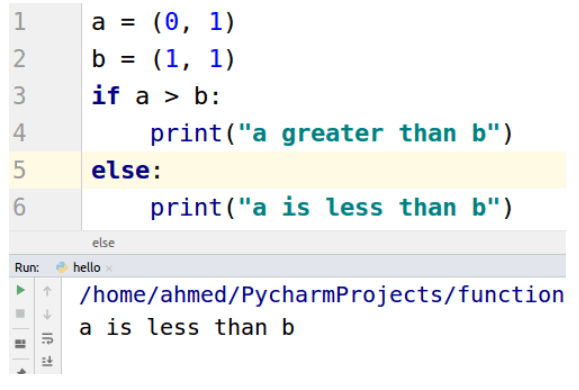Another example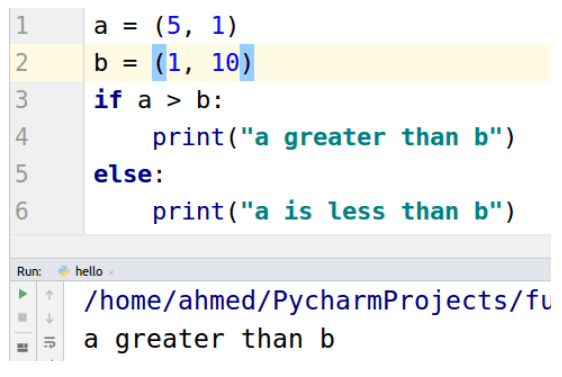## Slicing tuple into new tuple

We can use colon ‘:’ operator for performing slicing to make new tuples from originals. Let’s take a look at an example.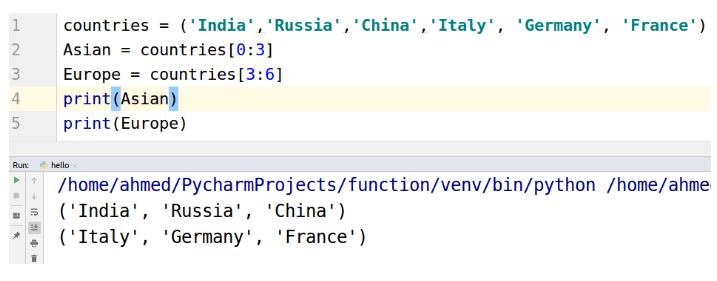## Deleting

You can not delete any value from tuple as tuples are immutable, but you can use del keyword to delete the object of tuple.